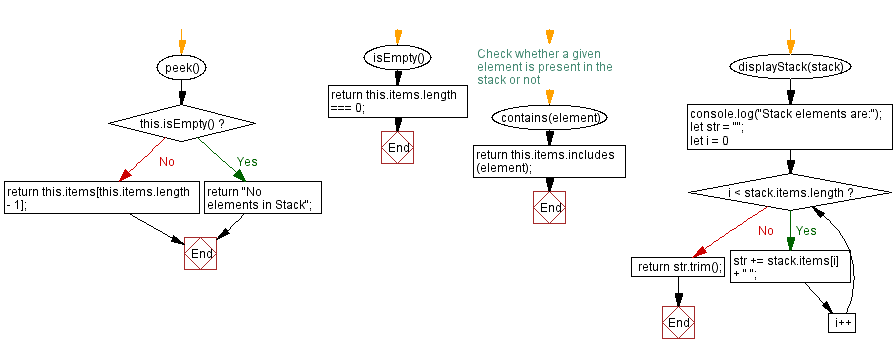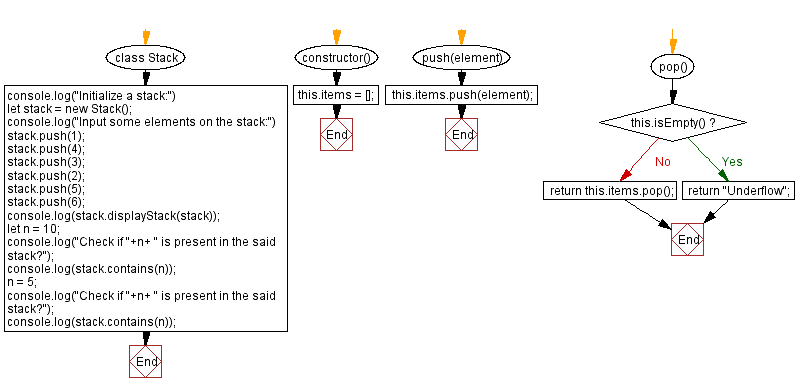# JavaScript Exercises: Check if an element is present or not in a stack

## JavaScript Stack: Exercise-8 with Solution

Write a JavaScript program to implement a stack that checks if a given element is present or not in the stack.

Sample Solution:

JavaScript Code:

``````class Stack {
constructor() {
this.items = [];
}

push(element) {
this.items.push(element);
}

pop() {
if (this.isEmpty())
return "Underflow";
return this.items.pop();
}

peek() {
if (this.isEmpty())
return "No elements in Stack";
return this.items[this.items.length - 1];
}

isEmpty() {
return this.items.length === 0;
}

// Check whether a given element is present in the stack or not
contains(element) {
return this.items.includes(element);
}
displayStack(stack) {
console.log("Stack elements are:");
let str = "";
for (let i = 0; i < stack.items.length; i++)
str += stack.items[i] + " ";
return str.trim();
}
}

console.log("Initialize a stack:")
let stack = new Stack();
console.log("Input some elements on the stack:")
stack.push(1);
stack.push(4);
stack.push(3);
stack.push(2);
stack.push(5);
stack.push(6);
console.log(stack.displayStack(stack));
let n = 10;
console.log("Check if "+n+ " is present in the said stack?");
console.log(stack.contains(n));
n = 5;
console.log("Check if "+n+ " is present in the said stack?");
console.log(stack.contains(n));
```
```

Sample Output:

```Initialize a stack:
Input some elements on the stack:
Stack elements are:
1 4 3 2 5 6
Check if 10 is present in the said stack?
false
Check if 5 is present in the said stack?
true
```

Flowchart:Live Demo:

See the Pen javascript-stack-exercise-6 by w3resource (@w3resource) on CodePen.

Improve this sample solution and post your code through Disqus

Stack Previous: Count all the elements in a given stack.
Stack Exercises Next: Remove duplicates from a given stack.

What is the difficulty level of this exercise?

Test your Programming skills with w3resource's quiz.

﻿